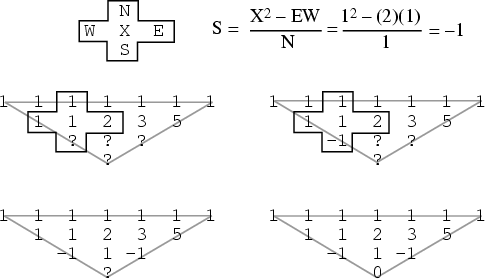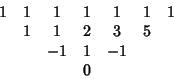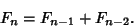## Quotient-Difference TableA quotient-difference table is a triangular Array of numbers constructed by drawing a sequence ofnumbers in a horizontal row and placing a 1 above each. An additional 1'' is then placed at the beginning and end of the row of 1s, and the value of rows underneath the original row is then determined by looking at groups of adjacent numbersand computingfor the elements falling within a triangle formed by the diagonals extended from the first and last 1,'' as illustrated above.

0s in quotient-difference tables form square windows'' which are bordered by Geometric Progressions. Quotient-difference tables eventually yield a row of 0s Iff the starting sequence is defined by a linear Recurrence Relation. For example, continuing the above example generated by the Fibonacci Numbersand it can be seen that a row of 0s emerges (and furthermore that an attempt to extend the table will result in division by zero). This verifies that the Fibonacci Numbers satisfy a linear recurrence, which is in fact given by the well-known formulaHowever, construction of a quotient-difference table for the Catalan Numbers, Motzkin Numbers, etc., does not lead to a row of zeros, suggesting that these numbers cannot be generated using a linear recurrence.